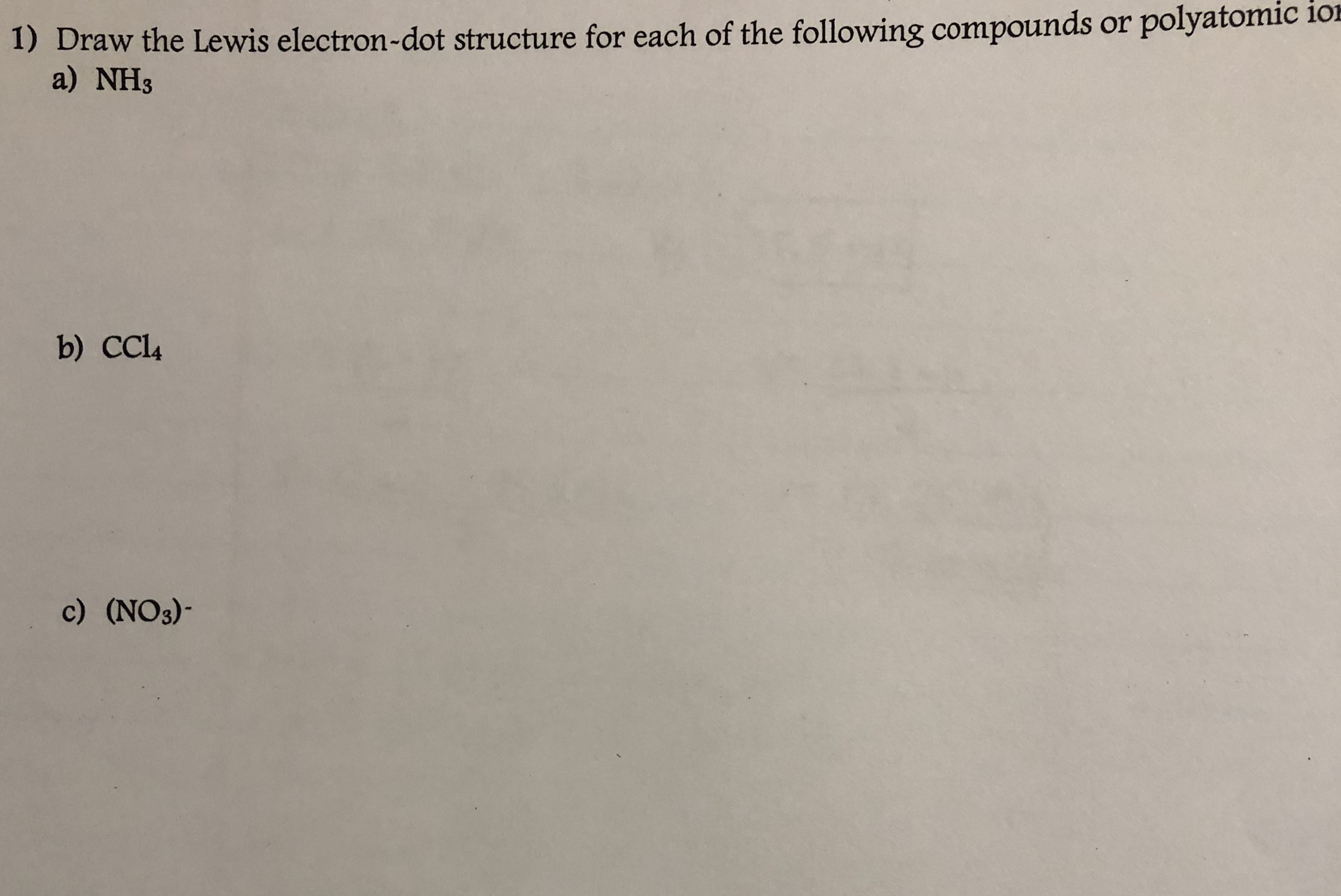the Lewis electron-dot structure for each of the following compounds or polyatomic ioa) NH3b) CCl4c) (NO3)-

Questionhelp_outlineImage Transcriptionclosethe Lewis electron-dot structure for each of the following compounds or polyatomic io a) NH3 b) CCl4 c) (NO3)- fullscreen
Step 1

Lewis structures are the diagrams that show the bonding between the atoms of the molecules and existing lone pairs of electrons.

Bonding electrons are those electrons which are shared between the atoms resulting in the formation of bond.

Non-bonding electrons are the valence electrons of the atom which are not shared with another atom.

Step 2

Part (a)

The rules for drawing the Lewis electron-dot structure of NH3

1. Calculate the total number of valence electrons in molecule :
Total number of valence electrons = valence electrons of N +3(valence electrons of H)

2. Arrange the atoms in such a way that the least electronegative atom is at the center. Then put the valence electrons around them such that each atom contributes at least 1 electron to single bond and the octet rule for each atom is followed.

3. To complete the octet of nitrogen and duet of hydrogen, each hydrogen, H atom forms single bond with nitrogen, N. The rest number of electrons are present as lone pair on nitrogen atom, the number of lone pair of electrons = Total number of valence electrons – Total number of electrons involved in bond formation.

The number of lone pair of electrons = 8 – 3 = 5 electrons

4. The central atom is N and on arranging all the valence electrons , we get the Lewis structure of NH as:

Step 3

Part (b)

The rules for drawing the Lewis electron-dot structure of CCl4 :

1. Calculate the total number of valence electrons in molecule :
Total number of valence electrons = valence electrons of C +4(valence electrons of Cl)

2. Arrange the atoms in such a way that the least electronegative atom is at the center. Then put the valence electrons around them such that each atom contributes at least 1 electron to single bond and the octet rule for each atom is followed.

3. To  complete the octet each chlorine, Cl atom forms si...

Want to see the full answer?

See Solution

Want to see this answer and more?

Our solutions are written by experts, many with advanced degrees, and available 24/7

See Solution
Tagged in

Chemistry